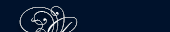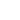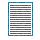НОВОСТИ   БИБЛИОТЕКА   ЭНЦИКЛОПЕДИЯ   КАРТА САЙТА   ССЫЛКИ   О САЙТЕ### THE WHOLE NUMBERS

Generally when numbers are written the numerals are grouped by threes so that it becomes easy for the eye to distinguish them.

Thus five million six hundred seventy-five thousand four hundred ninety-two is written as 5675492.

Often the groups of threes are separated from one another by commas, thus: 5,675,492.

Numbers, when written, are often described by the number of numerals they contain, the number of places. Thus 72 is a two-place number and 4895 is a four-place number.

Four-place numbers, especially dates, are often written without commas or spaces, as 1905, 1943.

#### Problems

1. Write the smallest three-place number.

2. Write the largest three-place number.

The addition of two or more numbers is an arithmetic operation by means of which a new number is obtained. This new number contains as many units as are contained in all of added numbers taken together. The numbers that are added are known as the addends.

The number resulting from the addition of two or more numbers is known as the sum. The sign for addition is + (plus).ПОИСК: